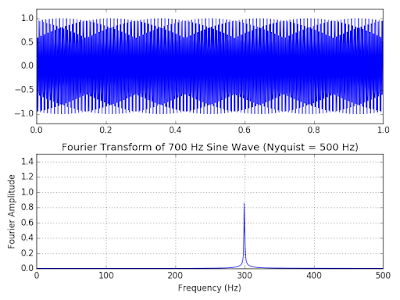## Tuesday, February 6, 2018

### Fourier Transform Talk and Python Code

————— 6 Feb 2018 —————

Lecture notes from the Fourier Transform brown bag talk:

————— 5 Feb 2018 —————

Well, it was probably a blur, but we got through a few good ideas. Got this from Max, who organizes the brown-bag lecture series. The video he links is, indeed, excellent. Thanks, Max!

Dr. Liner,
Thanks again for doing Brown Bag! Sorry it cut short, I had forgotten there was a a lab in their at 1:30 and didn't inform you.
Also, if you have time you should check out this introduction to Fourier Transforms: https://www.youtube.com/watch?v=spUNpyF58BY . It has a great intuitive visualization, and may be useful for sending to students who are learning FTs!
Best,
Max

————— 1 Feb 2018 —————

I'll be giving an improv brown-bag lecture next Monday on campus, here is the flyer.  Should be fun!

Cartoon creditFig 1. FFT of 50 Hz sine wave sampled at 1 millisecond (0.001 sec). Lower plot is the Fourier Transform amplitude spectrum.Fig 2. FFT of 50 Hz square wave showing harmonics. The harmonics arise because the Fourier Transform decomposes the signal into sine and cosine waves that are not a natural fit for square waves. To represent the square wave no singe frequency will suffice, it takes a doubly periodic family of sin-cos waves: each sin-cos is periodic in itself and the harmonics are periodic multiples. In the case shown, the nth harmonic (fn) is related to the fundamental (f0) by the rule: fn = f0*(2n+1) where n=0,1,2,...  Consequently, it is better practice to describe such signals as 50 cycles per second (cps) and reserve the term Hertz (Hz) for smooth signals. In passing, I note that harmonics can also arise from smooth signals if there is amplitude asymetry present, indicating nonlinear processes are at work. For discussion of this case, see Chapter 2 of my DISC book available from SEG or Amazon.
---------------------

Python FFT code, most of it is for pretty plotting.

import numpy as np
import matplotlib.pyplot as plt
import scipy.fftpack
from scipy import signal

# Number of sample points
nt = 1000
# sample spacing (seconds)
dt = 0.001
# make the time vector
t = np.linspace(0.0, nt*dt, nt)
# calculate sine function at each time point
y1 = np.sin(50.0 * 2.0*np.pi*t)
# do the FFT
yf = scipy.fftpack.fft(y1)
# make the frequency vector from zero to Nyquist
xf = np.linspace(0.0, 1.0/(2.0*dt), nt/2)

# plot the sine wave and its Fourier amplitude spectrum
plt.subplot(2,1,1)
plt.plot(t,y1)
plt.subplot(2,1,2)
plt.plot(xf, 2.0/nt * np.abs(yf[0:nt/2]))
plt.grid()
plt.ylim(0,1.5)
plt.title('Fourier Transform of 50 Hz Sine Wave')
plt.xlabel('Frequency (Hz)')
plt.ylabel('Fourier Amplitude')
plt.tight_layout()
plt.show()

# calculate the square wave function at each time point
y2 = signal.square(2 * np.pi * 50 * t)
yf = scipy.fftpack.fft(y2)

# plot the square wave and its Fourier amplitude spectrum
plt.subplot(2,1,1)
plt.plot(t,y2)
plt.subplot(2,1,2)
plt.plot(xf, 2.0/nt * np.abs(yf[0:nt/2]))
plt.grid()
plt.ylim(0,1.5)
plt.title('Fourier Transform of 50 Hz Square Wave')
plt.xlabel('Frequency (Hz)')
plt.ylabel('Fourier Amplitude')
plt.tight_layout()
plt.show()Fig 3. The code above can be used to visualize the concept of aliasing. The Nyqvist frequency, Fn=1/(2*dt), is the highest frequency that can be reliably measured for a given time sample rate.  In this example the time sample rate is 0.001 sec, yielding a Nyqvist of Fn=1/(2*dt)=500 Hz. However, the input signal (upper) is a 700 Hz sine wave, a full 200 Hz above Nyqvist. The Fourier amplitude spectrum (lower) shows that the 700 Hz signal frequency is wrapped, or reflected across, the Nyqvist frequency to appear as aliased energy at 300 Hz.Anonymous said...

Dear Professor Liner,

Would it be possible to broadcast this lesson live to students from another countries?

Best regards

Kristian

Prof. Christopher L. Liner said...

Hi Kristian, let me look into it and see what I can do. If not live, then maybe a video. Thank you for asking.

Efrain Andres Laverde Mera said...

Hi professor Christopher. Thanks so much for the lesson. I agreed with Kristian.

Again, thanks for your time and cheers Fromm bogota-colombia